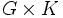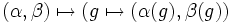# Direct product is cancellative for finite algebras in any variety with zero

## Statement

Suppose$\mathcal{V}$ is a variety of algebras that is a variety with zero. Suppose$G,H,K$ are finite algebras in$\mathcal{V}$. Further, suppose that the algebras$G \times H$ and$G \times K$ are isomorphic as algebras in$\mathcal{V}$. Then,$H$ is isomorphic to$K$.

## Facts used

1. Homomorphism set to direct product is Cartesian product of homomorphism sets: If$A,B,C$ are algebras, then there is a natural bijection:
•$\operatorname{Hom}(A,B) \times \operatorname{Hom}(A,C) \leftrightarrow \operatorname{Hom}(A,B \times C)$.
• The bijection is defined as:$(\alpha,\beta) \mapsto (g \mapsto (\alpha(g),\beta(g))$.
2. Homomorphism set is disjoint union of injective homomorphism sets: For algebras$A$ and$B$, let$\operatorname{Hom}(A,B)$ denotes the set of homomorphisms from$A$ to$B$, and$\operatorname{IHom}(A,B)$ denote the set of injective homomorphisms from$A$ to$B$. Then we have:$\operatorname{Hom}(A,B) = \bigsqcup_{~} \operatorname{IHom}(A/~, B)$.

Here$~$ varies over the set of all possible congruences on the algebra$A$.

## Proof

Given: Finite algebras$G, H, K$ such that$G \times H \cong G \times K$.

To prove:$H \cong K$.

Proof: Let$L$ be an arbitrary finite algebra in$\mathcal{V}$. Note that the trivial homomorphism refers to the map that sends every element to the zero element.

Step no. Assertion/construction Facts used Given data used Previous steps used Explanation
1$|\operatorname{Hom}(L,G \times H)| = |\operatorname{Hom}(L,G)||\operatorname{Hom}(L,H)|$ as an equality of finite numbers. Fact (1)$G,H$ are finite [SHOW MORE]
2$|\operatorname{Hom}(L,G \times K)| = |\operatorname{Hom}(L,G)||\operatorname{Hom}(L,K)|$ as an equality of finite numbers. Fact (1)$G,K$ are finite [SHOW MORE]
3$|\operatorname{Hom}(L,G \times H)| = |\operatorname{Hom}(L,G \times K)|$ as an equality of finite numbers.$G \times H \cong G \times K$ The number of homomorphisms to an algebra depends only on its isomorphism type.
4$|\operatorname{Hom}(L,H)| = |\operatorname{Hom}(L,K)|$ as an equality of finite numbers. Steps (1),(2),(3) [SHOW MORE]
5 For any finite algebra$L$, the number of injective homomorphisms from$L$ to$H$ equals the number of injective homomorphisms from$L$ to$K$. We show this by induction on the order of$L$. In other words,$|\operatorname{IHom}(L,H)| = |\operatorname{IHom}(L,K)|$ Fact (2) Step (4) [SHOW MORE]
6$H$ is isomorphic to a subalgebra of$K$ and$K$ is isomorphic to a subalgebra of$H$$H,K$ are finite Step (5) [SHOW MORE]
7$H$ is isomorphic to$K$$H,K$ are finite Step (6) [SHOW MORE]